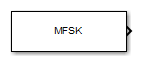# MFSK Waveform

Multiple frequency shift keying (MFSK) continuous waveform

• Library:
• Phased Array System Toolbox / Waveforms

•## Description

The MFSK Waveform block generates a multiple frequency-shift keying (MFSK) continuous waveform with a specified step time, sweep bandwidth, frequency offset, and number of steps. The block outputs an integer number of samples, steps, or sweeps. For details on the structure of an MFSK waveform, see `phased.MFSKWaveform`.

## Ports

### Output

expand all

MFSK waveform, returned as a complex-valued column vector containing the waveform samples.

• If the Output signal format parameter is set to `Steps`, the output of the port consists of the number of steps set by the Number of frequency steps in output parameter.

• If the Output signal format parameter is set to `Samples`, the output of the port consists of the number of samples set by the Number of samples in output parameter.

• If the Output signal format parameter is set to `Sweeps`, the output of the port consists of the number of sweeps set by the Number of sweeps in output parameter.

## Parameters

expand all

Sample rate of the signal, specified as a positive scalar. Units are in hertz.

Bandwidth of the MFSK sweep, specified as a positive scalar. Units are in hertz.

Time duration of each frequency step, specified as a positive scalar. Units are in seconds.

Total number of steps in each sweep, specified as an even positive integer.

Chirp offset frequency, specified as a real scalar. Units are in hertz. The offset determines the frequency translation between the two sequences.

Format of the output signal, specified as one of the following:

• `Steps` — The block outputs the number of samples contained in an integer number of frequency steps specified by the Number of frequency steps in output parameter.

• `Samples` — The block outputs the number of samples specified in the Number of samples in output parameter.

• `Sweeps` — The block outputs the number of samples contained in an integer number of sweeps specified by the Number of sweeps in output parameter.

Number of sweeps in the block output, specified as a positive integer.

#### Dependencies

This parameter appears only when you set Output signal format to `Sweeps`.

Number of steps in the block output, specified as a positive integer.

#### Dependencies

This parameter appears only when you set Output signal format to `Steps`.

Number of samples in the block output, specified as a positive integer.

Example: `1000`

#### Dependencies

To enable this parameter, set the Output signal format parameter to `Samples`.

Data Types: `double`

Block simulation, specified as `Interpreted Execution` or `Code Generation`. If you want your block to use the MATLAB® interpreter, choose `Interpreted Execution`. If you want your block to run as compiled code, choose ```Code Generation```. Compiled code requires time to compile but usually runs faster.

Interpreted execution is useful when you are developing and tuning a model. The block runs the underlying System object™ in MATLAB. You can change and execute your model quickly. When you are satisfied with your results, you can then run the block using ```Code Generation```. Long simulations run faster with generated code than in interpreted execution. You can run repeated executions without recompiling, but if you change any block parameters, then the block automatically recompiles before execution.

This table shows how the Simulate using parameter affects the overall simulation behavior.

When the Simulink® model is in `Accelerator` mode, the block mode specified using Simulate using overrides the simulation mode.

Acceleration Modes

 Block Simulation Simulation Behavior `Normal` `Accelerator` `Rapid Accelerator` `Interpreted Execution` The block executes using the MATLAB interpreter. The block executes using the MATLAB interpreter. Creates a standalone executable from the model. `Code Generation` The block is compiled. All blocks in the model are compiled.